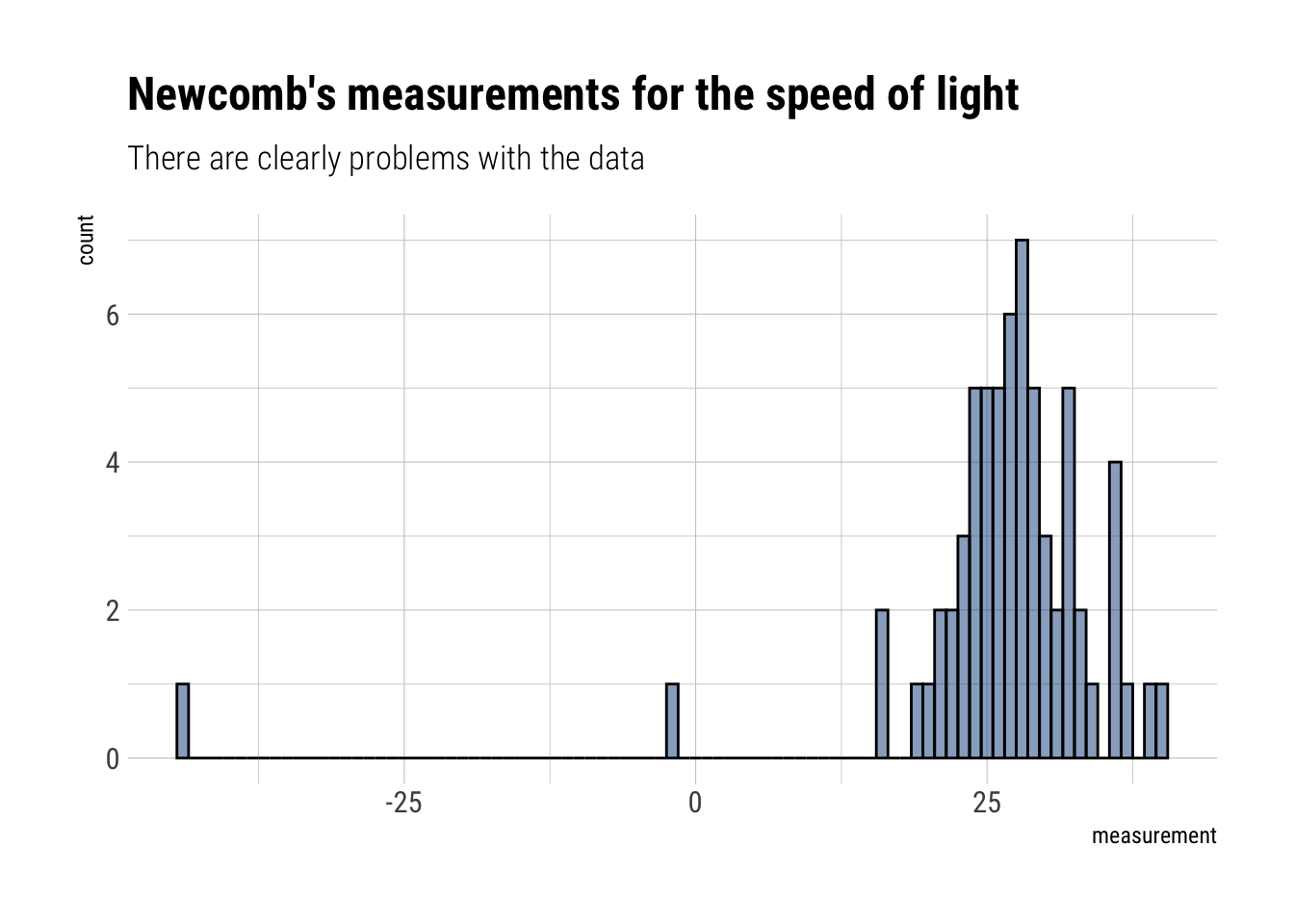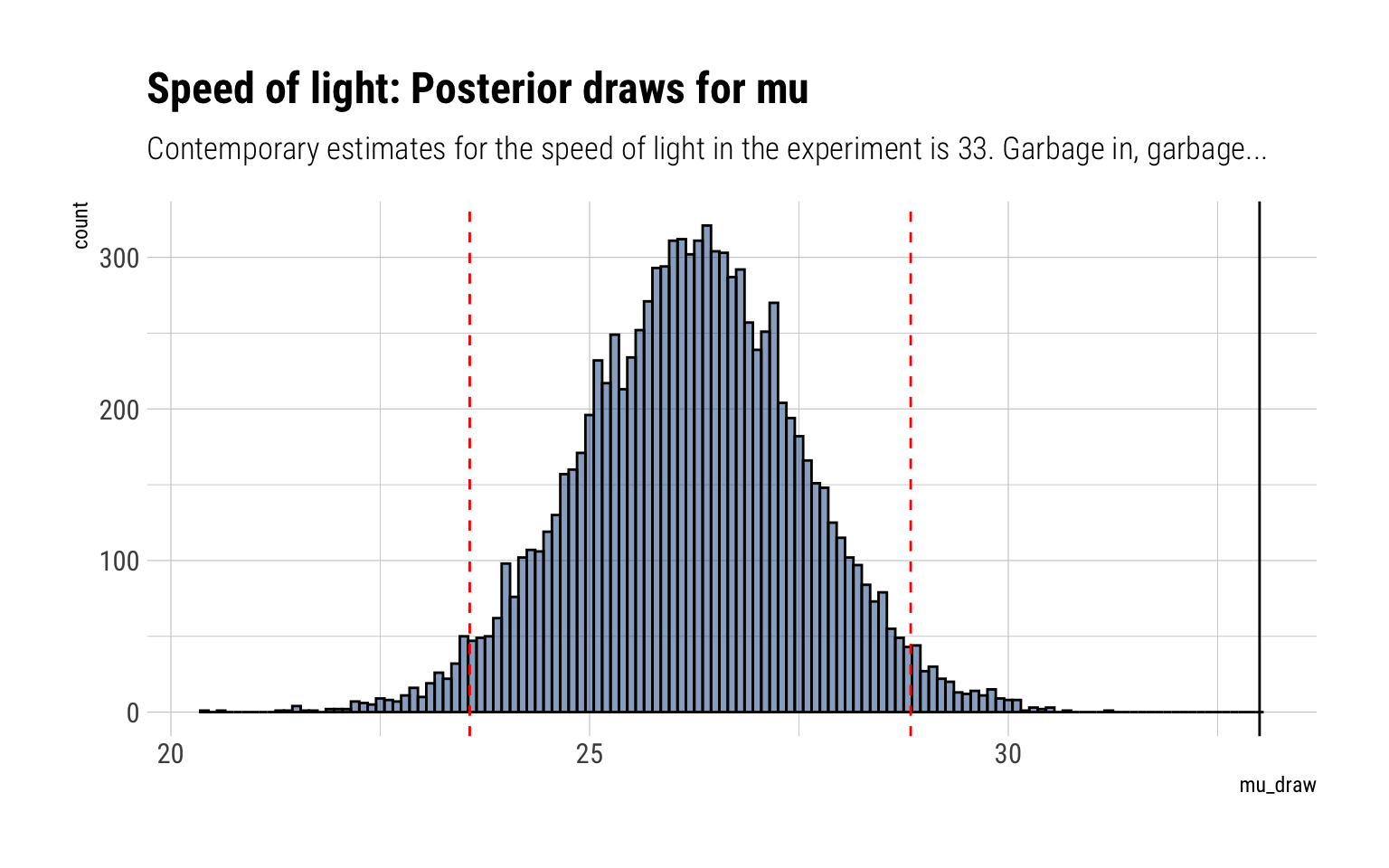# Bayesian Data Analysis: Week 3 -> Fitting a Gaussian probability model

Published

June 24, 2020

Bayesian Data Analysis (Gelman, Vehtari et. alter) is equals part a great introduction and THE reference for advanced Bayesian Statistics. Luckily, it’s freely available online. To make things even better for the online learner, Aki Vehtari (one of the authors) has a set of online lectures and homeworks that go through the basics of Bayesian Data Analysis.

Instead of going through the homeworks (due to the fear of ruining the fun for future students of Aki’s), I’ll go through some of the examples of the book as case studies. In this blogpost, I’ll (wrongly) estimate the speed of light from the measurements of Simon Newcomb’s 1882 experiments.

## The Gaussian Probability model

When fitting a Gaussian probability model, there are to parameters to estimate: $$\mu, \sigma$$. Therefore, we arrive at a joint posterior distribution:

$y | \mu, \sigma^2 \sim N(\mu, \sigma^2) \\ p(\mu, \sigma | y) \propto p (y | \mu, \sigma) p(\mu, \sigma)$ In this case, $$\sigma$$ is a nuisance parameter: we are only really interested in knowing $$\mu$$. The following we assume the non-informative prior:

$p(\mu, \sigma^2) \propto (\sigma^2)^{-1}$

### Posterior marginal of sigma

We can show that the marginal posterior distribution for $$\sigma$$ is:

$\sigma^2 | y \sim Inv -\chi^2 (n - 1, s^2)$ Where $$s^2$$ is the sample variance of the $$y_i$$’s.

### Marginal Conditional posterior for mu

Then:

$\mu | \sigma^2, y \sim N(\bar y, \sigma^2 / n)$ > The posterior distribution of $$\mu$$ can be regarded as a mixture of normal distributions, mixed over the scaled inverse $$\chi^2$$ distribution for the variance, $$\sigma^2$$.

### Marginal posterior for mu

$\dfrac{\mu - \bar y}{s/\sqrt{n}} | y \sim t_{n-1}$

Which has a nice correspondence with the distribution used for the mean estimator in frequentist statistics in the case of small samples.
## Fitting it with data

Then, let’s read the measurements from Newcomb’s experiment:

read_delim("http://www.stat.columbia.edu/~gelman/book/data/light.asc",
delim = " ", skip = 3,col_names = F) %>%
pivot_longer(everything()) %>%
select(value) %>%
drop_na()-> measurements

measurements %>%
ggplot(aes(value)) +
geom_histogram(binwidth = 1, color = "black", fill = "dodgerblue4", alpha = 0.5) +
labs(title = "Newcomb's measurements for the speed of light",
subtitle = "There are clearly problems with the data",
x = "measurement")There are clearly some problems with the data. Garbage in, garbage out.

### Marginal for sigma

We therefore can derive the marginal distribution for $$\sigma$$:

measurements%>%
skimr::skim()
 Name Piped data Number of rows 66 Number of columns 1 _______________________ Column type frequency: numeric 1 ________________________ Group variables None

Variable type: numeric

skim_variable n_missing complete_rate mean sd p0 p25 p50 p75 p100 hist
value 0 1 26.21 10.75 -44 24 27 30.75 40 ▁▁▁▂▇

Thus:

$\sigma^2 | y \sim Inv -\chi^2 (65, 10.7^2)$

Whereas for mu we have :

$\dfrac{\mu - 26.2}{10.7/\sqrt{66}} | y \sim t_{n-1}$ In code:

rsinvchisq <- function(n, nu, s2, ...) nu*s2 / rchisq(n , nu, ...)

sigma_draw = rinvchisq(1000, 65, 10.7^2)
mu_draw = rtnew(10000, df = 65, mean = 26.2, scale = 10.7/sqrt(66) )

The 95% credible interval for our $$\mu$$ is thus:

interval <- rethinking::PI(mu_draw, prob = 0.95)
lower <- interval[]
upper <- interval[]
glue::glue("The 95% credible interval is thus: {round(lower, 1)}, {round(upper, 1)}")
The 95% credible interval is thus: 23.6, 28.8

A visualization may help:

data.frame(mu_draw) %>%
ggplot(aes(mu_draw)) +
geom_histogram(binwidth = 0.1, color = "black", fill = "dodgerblue4", alpha = 0.5) +
geom_vline(aes(xintercept = lower), color = "red", linetype = 2) +
geom_vline(aes(xintercept = upper), color = "red", linetype = 2) +
geom_vline(aes(xintercept = 33), color = "black", linetype = 1) +
labs(title = "Speed of light: Posterior draws for mu",
subtitle = "Contemporary estimates for the speed of light in the experiment is 33. Garbage in, garbage...")